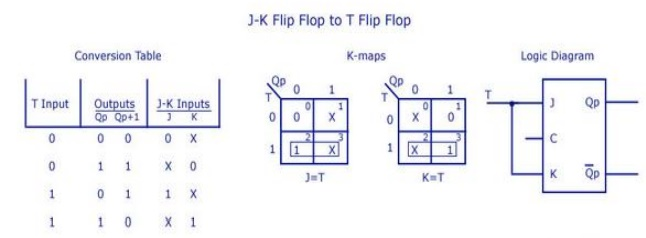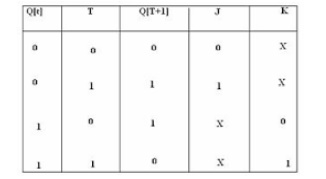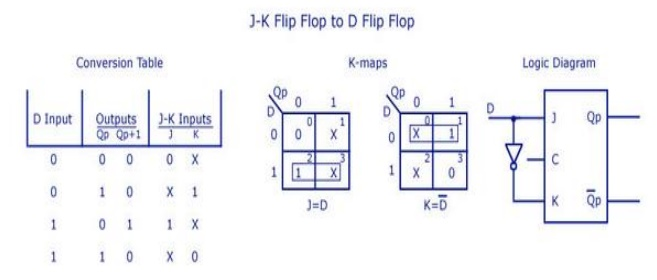Home | | Digital Electronics | Important Short Questions and Answers: Sequential Circuits

# Important Short Questions and Answers: Sequential Circuits

Digital Electronics - Sequential Circuits - Important Short Questions and Answers: Sequential Circuits

SEQUENTIAL CIRCUITS

1.         Mention any two differences between the edge triggering and level triggering.

Level Triggering:

1)          The input signal is sampled when the clock signal is either HIGH or LOW.

2)          It is sensitive to Glitches.

Example: Latch.

Edge Triggering:

1)          The input signal is sampled at the RISING EDGE or FALLING EDGE of the clock signal.

2)          It is not-sensitive to Glitches.

Example: Flipflop.

2.         What is meant by programmable counter? Mention its application.

·              A counter that divides an input frequency by a number which can be programmed into decades of synchronous down counters.

·              Decades, with additional decoding and control logic, give the equivalent of a divide-by N counter system, where N can be made equal to any number.

Appication:

·              Microprocessor.

·              Traffic light controller.

·              Street light controller.

3.         Write the characteristic equation of a JK flip-flop.

The characteristic equation of a JK flip-flop is given by

Q(next) = JQ' + K'Q

4.         State the differences between Moore and mealy state machine.

1)Mealy Machines tend to have less states

a)           Different outputs on arcs (n^2) rather than states (n).

2)          Moore Machines are safer to use

a)           Outputs change at clock edge (always one cycle later).

b)          In Mealy machines, input change can cause output change as soon as logic is done

– a big problem when two machines are interconnected asynchronous feedback. 3) Mealy Machines react faster to inputs

b)          React in same cycle – don't need to wait for clock.

c)           In Moore machines, more logic may be necessary to decode state into outputs – more gate delays after.

5. Realise T-FF from JK-FF.6. Convert JK flip-flop to T flip-flop.7.           How many flip-flops are required to build a binary counter that counts from 0 to 1023?

If the number of flip-flops required is n, then

2n-1=1023

n=10 since 210=1024

8.           Compare the logics of synchronous counter and ripple counter.

Asynchronous counter:

1.           In this type of counter flipflop are connected in such a way that output of first flip-flop drives the clock for next flip-flop.

2.           All the flip-flop are not clocked simultaneously.

3.           Logic circuit is very simple even for more number of states.

synchronous counter:

1.           In this type there is no connection between output of first flip-flop and clock input of the next flip-flop.

2.           All the flip-flop are clocked simultaneously.

9. Sketch the logic diagram of a clocked SR flip-flop.10.      How do you eliminate the race around condition in a JK flip-flop?

·              When the input to the JK flip-flop is j=1 and k=1, the race around condition occurs, i.e it occurs when the time period of the clock pulse is greater than the propagation delay of the flip flop.

·              the output changes or toggles in a single clock period. If it toggles even number of times the output is same but if it toggles odd number of times then the output is complimented.

To avoid race around condition we cant make the clock pulse smaller than the propagation delay so we use

1.           Master slave JK flip flop

2.           Positive or negative edge triggering

11. Draw the state table and excitation table of T flip-flop.12.      A 4-bit binary ripple counter is operated with clock frequency of 1KHz. What is the output frequency of its third Flip flop?

The output frequency of third flip-flop is: ½3=1/8KHz.

13. Realize JK flip-flop using D flip-flop.14.    Design a 3-bit ring counter and find the mod of the designed counter.15.    Define latches.

Latch is a simple memory element, which consists of a pair of logic gates with their inputs and outputs inter connected in a feedback arrangement, which permits a single bit to be stored.

16.    Write short notes on Digital Clock.

A digital clock is a simplified logic diagram of a digital clock that displays seconds, minutes, and hours. First, a 60 Hz sinusoidal ac voltage is converted to a 60 Hz pulse waveform and divided own to a 1Hz pulse waveform by a divide-by-60 counter formed by a divide-by-10 counter allowed by a divide-by-6 counter. Both the seconds and minutes counts are also produced by divide-by-60 counters.

Study Material, Lecturing Notes, Assignment, Reference, Wiki description explanation, brief detail
Digital Electronics : Sequential Circuits : Important Short Questions and Answers: Sequential Circuits |